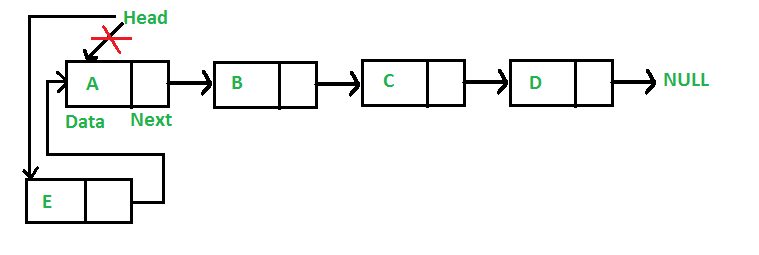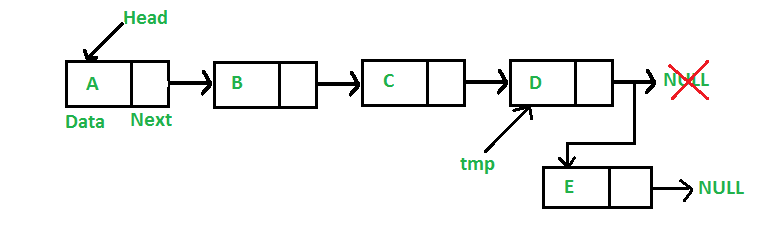# C++ Program For Inserting A Node In A Linked List

• Last Updated : 22 Dec, 2021

We have introduced Linked Lists in the previous post. We also created a simple linked list with 3 nodes and discussed linked list traversal.
All programs discussed in this post consider the following representations of the linked list.

## C++

 `// A linked list node ``class` `Node ``{ ``    ``public``:``    ``int` `data; ``    ``Node *next; ``}; ``// This code is contributed by rathbhupendra`

In this post, methods to insert a new node in linked list are discussed. A node can be added in three ways
1) At the front of the linked list
2) After a given node.
3) At the end of the linked list.

Add a node at the front: (4 steps process)Following are the 4 steps to add a node at the front.

## C++

 `/* Given a reference (pointer to pointer) ``   ``to the head of a list and an int, ``   ``inserts a new node on the front of ``   ``the list. */``void` `push(Node** head_ref, ``          ``int` `new_data) ``{ ``    ``// 1. Allocate node ``    ``Node* new_node = ``new` `Node(); `` ` `    ``// 2. Put in the data ``    ``new_node->data = new_data; `` ` `    ``// 3. Make next of new node ``    ``//    as head ``    ``new_node->next = (*head_ref); `` ` `    ``// 4. Move the head to point to ``    ``//    the new node ``    ``(*head_ref) = new_node; ``} ``// This code is contributed by rathbhupendra`

Time complexity of push() is O(1) as it does a constant amount of work.
Add a node after a given node: (5 steps process)
We are given a pointer to a node, and the new node is inserted after the given node.

## C++

 `// Given a node prev_node, insert a ``// new node after the given prev_node``void` `insertAfter(Node* prev_node, ``int` `new_data)  ``{  ``    ``// 1. Check if the given prev_node ``    ``//    is NULL ``    ``if` `(prev_node == NULL)  ``    ``{  ``        ``cout << ``"the given previous node cannot be NULL"``;  ``        ``return``;  ``    ``} ``   ` `    ``// 2. Allocate new node``    ``Node* new_node = ``new` `Node(); ``   ` `    ``// 3. Put in the data ``    ``new_node->data = new_data;  ``   ` `    ``// 4. Make next of new node as ``    ``// next of prev_node ``    ``new_node->next = prev_node->next;  ``   ` `    ``// 5. move the next of prev_node``    ``// as new_node ``    ``prev_node->next = new_node;  ``} ``// This code is contributed by anmolgautam818`

Time complexity of insertAfter() is O(1) as it does a constant amount of work.

Add a node at the end: (6 steps process)
The new node is always added after the last node of the given Linked List. For example if the given Linked List is 5->10->15->20->25 and we add an item 30 at the end, then the Linked List becomes 5->10->15->20->25->30.
Since a Linked List is typically represented by the head of it, we have to traverse the list till the end and then change the next to last node to a new node.Following are the 6 steps to add node at the end.

## C++

 `// Given a reference (pointer to pointer) to ``// the head of a list and an int, appends a ``// new node at the end ``void` `append(Node** head_ref, ``int` `new_data)  ``{  ``   ` `    ``// 1. Allocate node ``    ``Node* new_node = ``new` `Node(); ``   ` `    ``// Used in step 5 ``    ``Node *last = *head_ref; ``   ` `    ``// 2. Put in the data ``    ``new_node->data = new_data;  ``   ` `    ``// 3. This new node is going to be  ``    ``//    the last node, so make next of  ``    ``//    it as NULL``    ``new_node->next = NULL;  ``   ` `    ``// 4. If the Linked List is empty, ``    ``//    then make the new node as head ``    ``if` `(*head_ref == NULL)  ``    ``{  ``        ``*head_ref = new_node;  ``        ``return``;  ``    ``}  ``   ` `    ``// 5. Else traverse till the last node ``    ``while` `(last->next != NULL)  ``        ``last = last->next;  ``   ` `    ``// 6. Change the next of last node ``    ``last->next = new_node;  ``    ``return``;  ``}  ``// This code is contributed by anmolgautam818`

Time complexity of append is O(n) where n is the number of nodes in the linked list. Since there is a loop from head to end, the function does O(n) work.
This method can also be optimized to work in O(1) by keeping an extra pointer to the tail of linked list/

Following is a complete program that uses all of the above methods to create a linked list.

## C++

 `// A complete working C++ program to demonstrate ``// all insertion methods on Linked List ``#include ``using` `namespace` `std;`` ` `// A linked list node ``class` `Node ``{ ``    ``public``:``    ``int` `data; ``    ``Node *next; ``}; `` ` `/* Given a reference (pointer to pointer)``   ``to the head of a list and an int, inserts``   ``a new node on the front of the list. */``void` `push(Node** head_ref, ``int` `new_data) ``{ ``    ``// 1. Allocate node ``    ``Node* new_node = ``new` `Node();`` ` `    ``// 2. Put in the data ``    ``new_node->data = new_data; `` ` `    ``// 3. Make next of new node as head``    ``new_node->next = (*head_ref); `` ` `    ``// 4. Move the head to point to the ``    ``//    new node ``    ``(*head_ref) = new_node; ``} `` ` `/* Given a node prev_node, insert a new ``   ``node after the given prev_node */``void` `insertAfter(Node* prev_node, ``                 ``int` `new_data) ``{ ``    ``/* 1. Check if the given prev_node ``          ``is NULL */``    ``if` `(prev_node == NULL) ``    ``{ ``        ``cout << ``"the given previous node cannot be NULL"``; ``        ``return``; ``    ``} `` ` `    ``// 2. Allocate new node ``    ``Node* new_node = ``new` `Node();`` ` `    ``// 3. Put in the data ``    ``new_node->data = new_data; `` ` `    ``// 4. Make next of new node as next ``    ``//    of prev_node ``    ``new_node->next = prev_node->next; `` ` `    ``// 5. Move the next of prev_node ``    ``//    as new_node ``    ``prev_node->next = new_node; ``} `` ` `/* Given a reference (pointer to pointer) ``   ``to the head of a list and an int, ``   ``appends a new node at the end */``void` `append(Node** head_ref, ``            ``int` `new_data) ``{ ``    ``// 1. Allocate node ``    ``Node* new_node = ``new` `Node();`` ` `    ``// Used in step 5``    ``Node *last = *head_ref; `` ` `    ``// 2. Put in the data ``    ``new_node->data = new_data; `` ` `    ``/* 3. This new node is going to be ``          ``the last node, so make next of ``          ``it as NULL */``    ``new_node->next = NULL; `` ` `    ``/* 4. If the Linked List is empty,``    ``then make the new node as head */``    ``if` `(*head_ref == NULL) ``    ``{ ``        ``*head_ref = new_node; ``        ``return``; ``    ``} `` ` `    ``// 5. Else traverse till the``    ``//    last node ``    ``while` `(last->next != NULL) ``        ``last = last->next; `` ` `    ``// 6. Change the next of last node ``    ``last->next = new_node; ``    ``return``; ``} `` ` `// This function prints contents of``// linked list starting from head ``void` `printList(Node *node) ``{ ``    ``while` `(node != NULL) ``    ``{ ``        ``cout<<``" "``<data; ``        ``node = node->next; ``    ``} ``} `` ` `// Driver code``int` `main() ``{ ``    ``// Start with the empty list ``    ``Node* head = NULL; ``     ` `    ``// Insert 6. So linked list ``    ``// becomes 6->NULL ``    ``append(&head, 6); ``     ` `    ``// Insert 7 at the beginning. ``    ``// So linked list becomes ``    ``// 7->6->NULL ``    ``push(&head, 7); ``     ` `    ``// Insert 1 at the beginning. ``    ``// So linked list becomes ``    ``// 1->7->6->NULL ``    ``push(&head, 1); ``     ` `    ``// Insert 4 at the end. So ``    ``// linked list becomes ``    ``// 1->7->6->4->NULL ``    ``append(&head, 4); ``     ` `    ``// Insert 8, after 7. So linked ``    ``// list becomes 1->7->8->6->4->NULL ``    ``insertAfter(head->next, 8); ``     ` `    ``cout << ``"Created Linked list is: "``; ``    ``printList(head); ``     ` `    ``return` `0; ``} ``// This code is contributed by rathbhupendra`

Output:

` Created Linked list is:  1  7  8  6  4`

Please refer complete article on Linked List | Set 2 (Inserting a node) for more details!

My Personal Notes arrow_drop_up